# Misc Physics Videos

Stop wasting time on Youtube. Browse our curated collection of amazing Misc Physics videos. We did all the work for you!### Symmetry and the Laws of Nature by Umesh Waghmare

• 0
• 0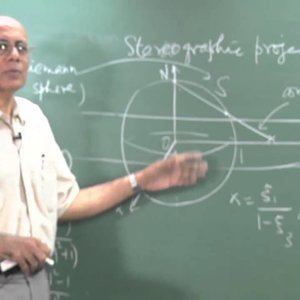### Mathematical Physics by Prof. V. Balakrishnan (NPTEL):- Lecture 01: Analytic functions of a complex variable (Part I)

• 1
• 0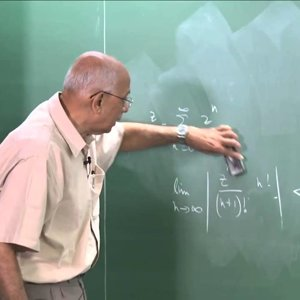### Mathematical Physics by Prof. V. Balakrishnan (NPTEL):- Lecture 02: Analytic functions of a complex variable (Part II)

• 1
• 0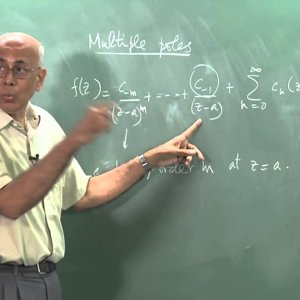### Mathematical Physics by Prof. V. Balakrishnan (NPTEL):- Lecture 03: Calculus of residues (Part I)

• 1
• 0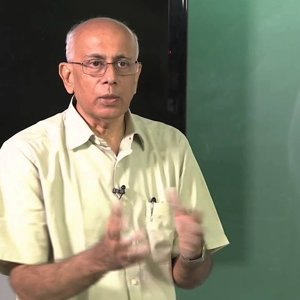### Mathematical Physics by Prof. V. Balakrishnan (NPTEL):- Lecture 04: Calculus of residues (Part II)

• 0
• 0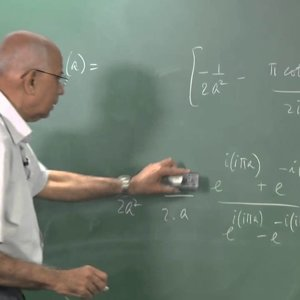### Mathematical Physics by Prof. V. Balakrishnan (NPTEL):- Lecture 05: Calculus of residues (Part III)

• 0
• 0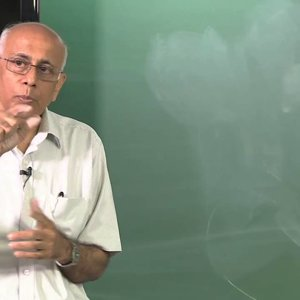### Mathematical Physics by Prof. V. Balakrishnan (NPTEL):- Lecture 06: Calculus of residues (Part IV)

• 0
• 0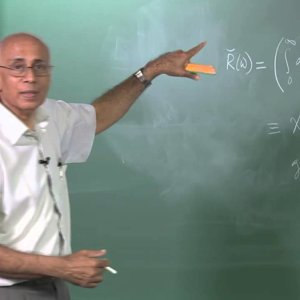### Mathematical Physics by Prof. V. Balakrishnan (NPTEL):- Lecture 07: Linear response; dispersion relations (Part I)

• 0
• 0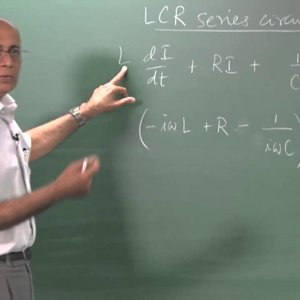### Mathematical Physics by Prof. V. Balakrishnan (NPTEL):- Lecture 08: Linear response; dispersion relations (Part II)

• 0
• 0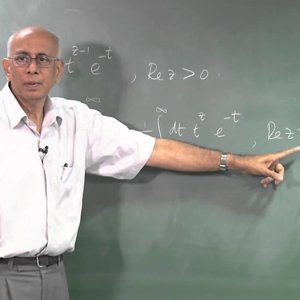### Mathematical Physics by Prof. V. Balakrishnan (NPTEL):- Lecture 09: Analytic continuation and the gamma function (Part I)

• 0
• 0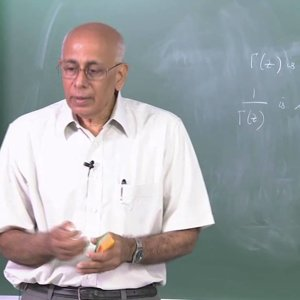### Mathematical Physics by Prof. V. Balakrishnan (NPTEL):- Lecture 10: Analytic continuation and the gamma function (Part II)

• 0
• 0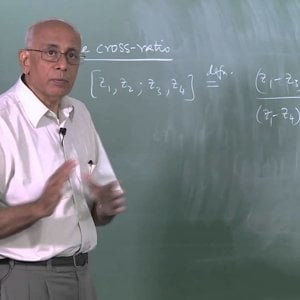### Mathematical Physics by Prof. V. Balakrishnan (NPTEL):- Lecture 11: Möbius transformations (Part I)

• 0
• 0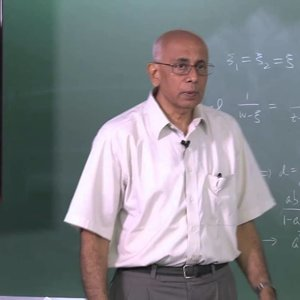### Mathematical Physics by Prof. V. Balakrishnan (NPTEL):- Lecture 12: Möbius transformations (Part II)

• 0
• 0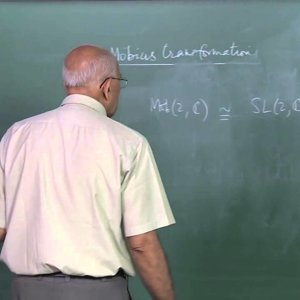### Mathematical Physics by Prof. V. Balakrishnan (NPTEL):- Lecture 13: Möbius transformations (Part III)

• 0
• 0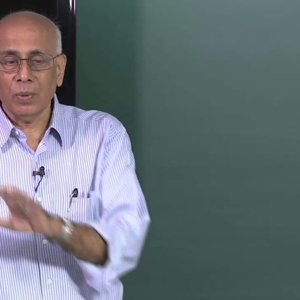### Mathematical Physics by Prof. V. Balakrishnan (NPTEL):- Lecture 14: Multivalued functions; integral representations (Part I)

• 0
• 0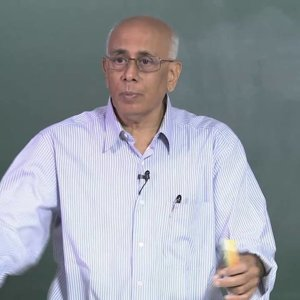### Mathematical Physics by Prof. V. Balakrishnan (NPTEL):- Lecture 15: Multivalued functions; integral representations (Part II)

• 0
• 0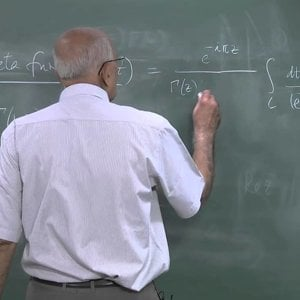### Mathematical Physics by Prof. V. Balakrishnan (NPTEL):- Lecture 16: Multivalued functions; integral representations (Part III)

• 0
• 0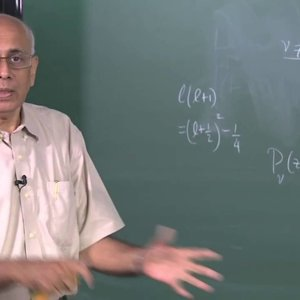### Mathematical Physics by Prof. V. Balakrishnan (NPTEL):- Lecture 17: Multivalued functions; integral representations (Part IV)

• 0
• 0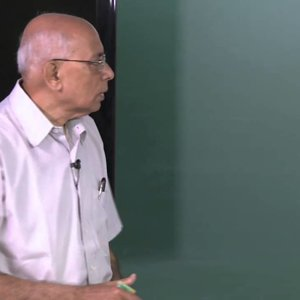### Mathematical Physics by Prof. V. Balakrishnan (NPTEL):- Lecture 18: Laplace transforms (Part I)

• 0
• 0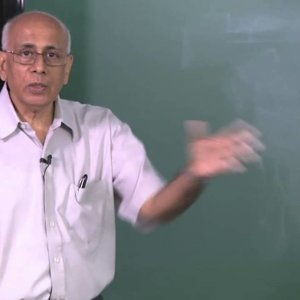### Mathematical Physics by Prof. V. Balakrishnan (NPTEL):- Lecture 19: Laplace transforms (Part II)

• 0
• 0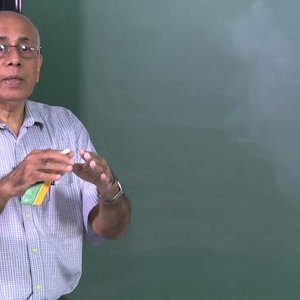### Mathematical Physics by Prof. V. Balakrishnan (NPTEL):- Lecture 20: Fourier transforms (Part I)

• 0
• 0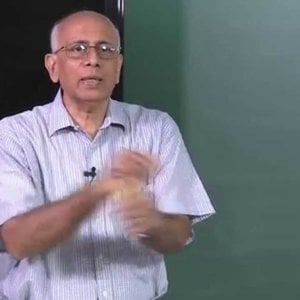### Mathematical Physics by Prof. V. Balakrishnan (NPTEL):- Lecture 21: Fourier transforms (Part II)

• 0
• 0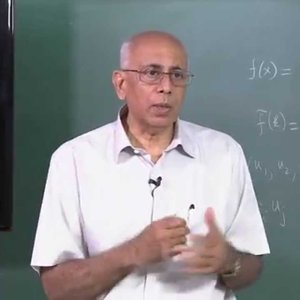### Mathematical Physics by Prof. V. Balakrishnan (NPTEL):- Lecture 22: Fourier transforms (Part III)

• 0
• 0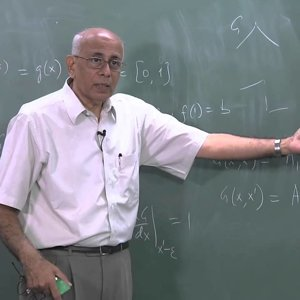### Mathematical Physics by Prof. V. Balakrishnan (NPTEL):- Lecture 23: Fundamental Green function for Δ2(Part I)

• 0
• 0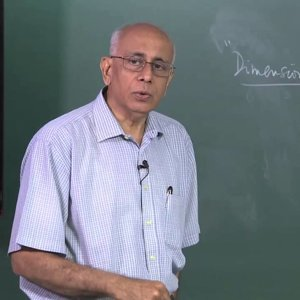### Mathematical Physics by Prof. V. Balakrishnan (NPTEL):- Lecture 24: Fundamental Green function for Δ2 (Part II)

• 0
• 0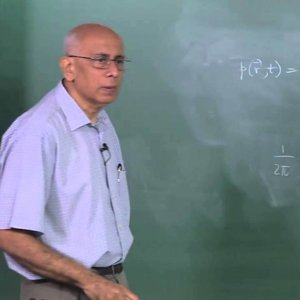### Mathematical Physics by Prof. V. Balakrishnan (NPTEL):- Lecture 25: The diffusion equation (Part I)

• 0
• 0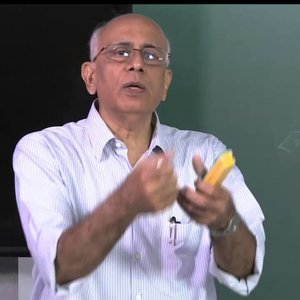### Mathematical Physics by Prof. V. Balakrishnan (NPTEL):- Lecture 26: The diffusion equation (Part II)

• 0
• 0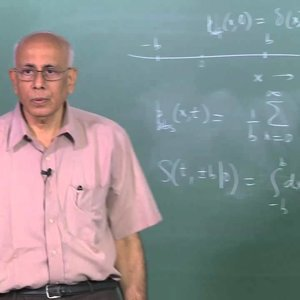### Mathematical Physics by Prof. V. Balakrishnan (NPTEL):- Lecture 27: The diffusion equation (Part III)

• 0
• 0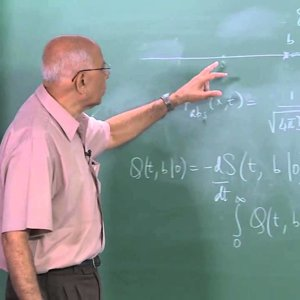### Mathematical Physics by Prof. V. Balakrishnan (NPTEL):- Lecture 28: The diffusion equation (Part IV)

• 0
• 0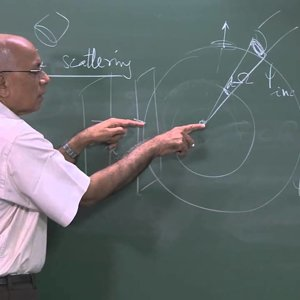### Mathematical Physics by Prof. V. Balakrishnan (NPTEL):- Lecture 29: Green function for (Δ2 + k2); nonrelativistic scattering (Part I)

• 0
• 0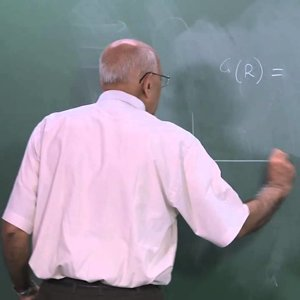### Mathematical Physics by Prof. V. Balakrishnan (NPTEL):- Lecture 30: Green function for (Δ2 + k2); nonrelativistic scattering (Part II)

• 0
• 0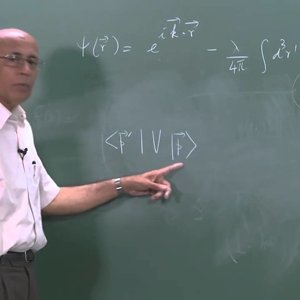### Mathematical Physics by Prof. V. Balakrishnan (NPTEL):- Lecture 31: Green function for (Δ2 + k2); nonrelativistic scattering (Part III)

• 0
• 0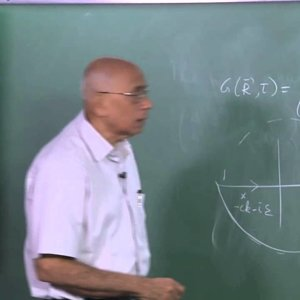### Mathematical Physics by Prof. V. Balakrishnan (NPTEL):- Lecture 32: The wave equation (Part I)

• 0
• 0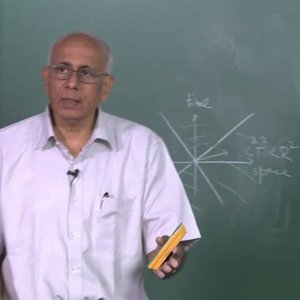### Mathematical Physics by Prof. V. Balakrishnan (NPTEL):- Lecture 33: The wave equation (Part II)

• 0
• 0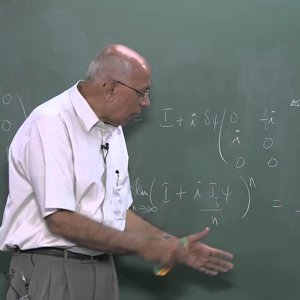### Mathematical Physics by Prof. V. Balakrishnan (NPTEL):- Lecture 34: The rotation group and all that (Part I)

• 0
• 0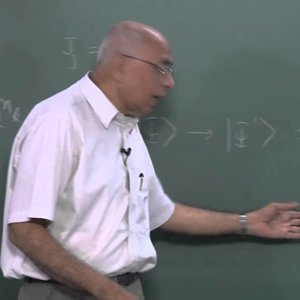### Mathematical Physics by Prof. V. Balakrishnan (NPTEL):- Lecture 35: The rotation group and all that (Part II)

• 0
• 0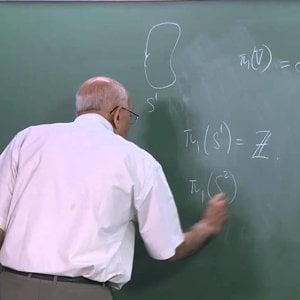### Mathematical Physics by Prof. V. Balakrishnan (NPTEL):- Lecture 36: The rotation group and all that (Part III)

• 0
• 0### Mathematical Physics 1 by Prof. Samudra Roy (NPTEL):- Introduction

• 0
• 0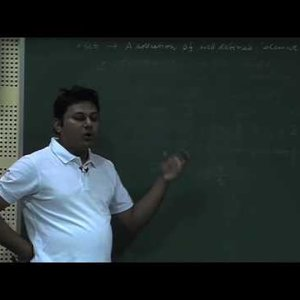### Mathematical Physics 1 by Prof. Samudra Roy (NPTEL):- Lecture 1 : Set, Group, Field, Ring

• 0
• 0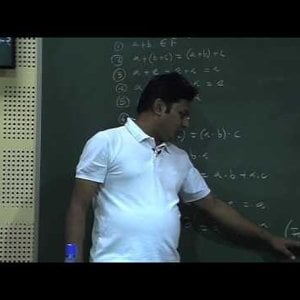### Mathematical Physics 1 by Prof. Samudra Roy (NPTEL):- Lecture 2 : Vector Space

• 0
• 0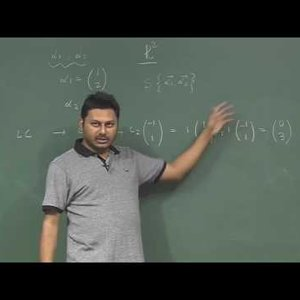### Mathematical Physics 1 by Prof. Samudra Roy (NPTEL):- Lecture 3 : Span, Linear combination of vectors

• 0
• 0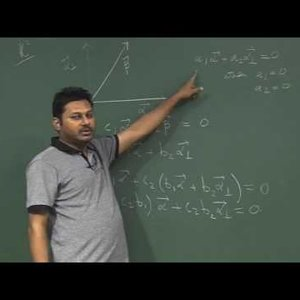### Mathematical Physics 1 by Prof. Samudra Roy (NPTEL):- Lecture 4 : Linearly dependent and independent vector, Basis

• 0
• 0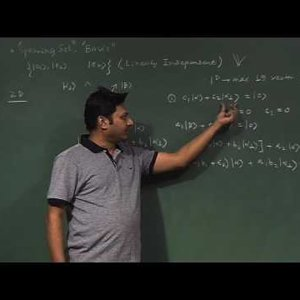### Mathematical Physics 1 by Prof. Samudra Roy (NPTEL):- Lecture 5 : Dual Space

• 0
• 0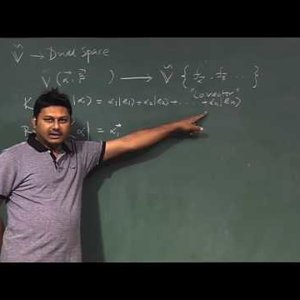### Mathematical Physics 1 by Prof. Samudra Roy (NPTEL):- Lecture 6 : Inner Product

• 0
• 0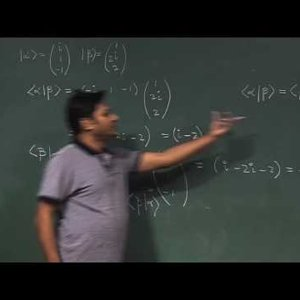### Mathematical Physics 1 by Prof. Samudra Roy (NPTEL):- Lecture 7 : Schwarz Inequality

• 0
• 0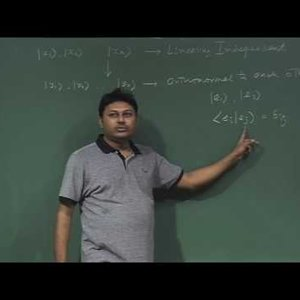### Mathematical Physics 1 by Prof. Samudra Roy (NPTEL):- Lecture 8 : Inner product space, Gram- Schmidt Ortho-normalization

• 0
• 0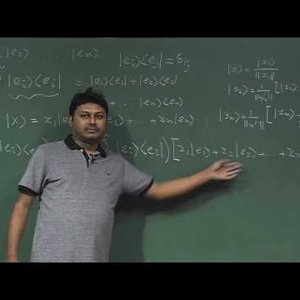### Mathematical Physics 1 by Prof. Samudra Roy (NPTEL):- Lecture 9 : Projection operator

• 0
• 0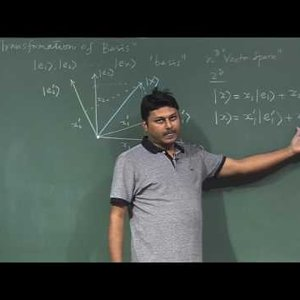### Mathematical Physics 1 by Prof. Samudra Roy (NPTEL):- Lecture 10: Transformation of Basis

• 0
• 0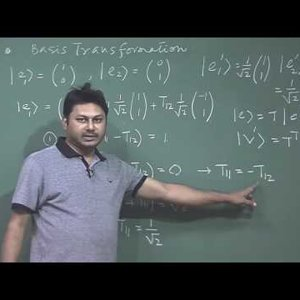### Mathematical Physics 1 by Prof. Samudra Roy (NPTEL):- Lecture 11: Transformation of Basis (Continue)

• 0
• 0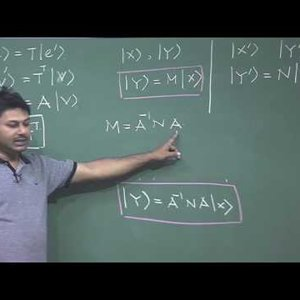### Mathematical Physics 1 by Prof. Samudra Roy (NPTEL):- Lecture 12: Unitary transformation, Similarity Transformation

• 0
• 0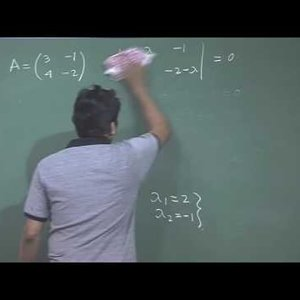### Mathematical Physics 1 by Prof. Samudra Roy (NPTEL):- Lecture 13: Eigen Value, Eigen Vectors

• 0
• 0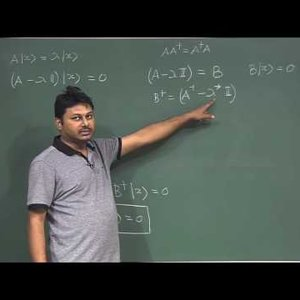### Mathematical Physics 1 by Prof. Samudra Roy (NPTEL):- Lecture 14: Normal Matrix

• 0
• 0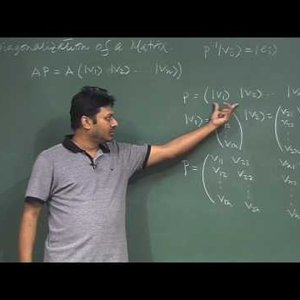### Mathematical Physics 1 by Prof. Samudra Roy (NPTEL):- Lecture 15: Diagonalization of a Matrix

• 0
• 0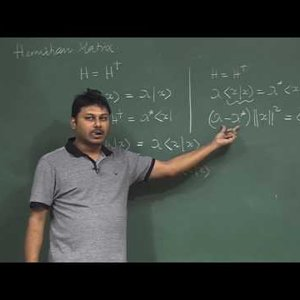### Mathematical Physics 1 by Prof. Samudra Roy (NPTEL):- Lecture 16: Hermitian Matrix

• 0
• 0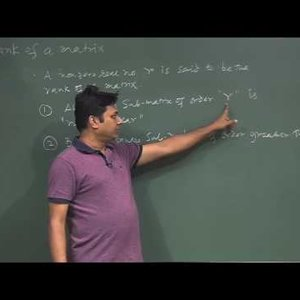### Mathematical Physics 1 by Prof. Samudra Roy (NPTEL):- Lecture 17 : Rank of a Matrix

• 0
• 0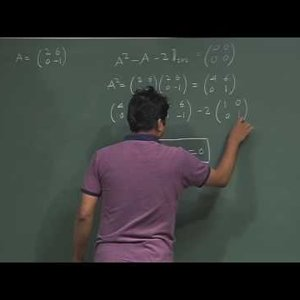### Mathematical Physics 1 by Prof. Samudra Roy (NPTEL):- Lecture 18 : Cayley - Hamilton Theorem, Function space

• 0
• 0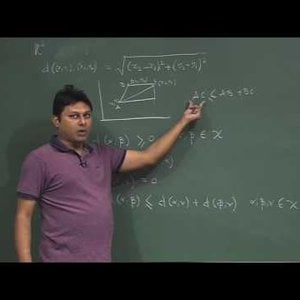### Mathematical Physics 1 by Prof. Samudra Roy (NPTEL):- Lecture 19: Metric Space, Linearly dependent –independent functions

• 0
• 0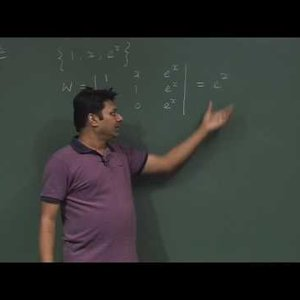### Mathematical Physics 1 by Prof. Samudra Roy (NPTEL):- Lecture 20 : Linearly dependent –independent functions (Cont), Inner Product of functions

• 0
• 0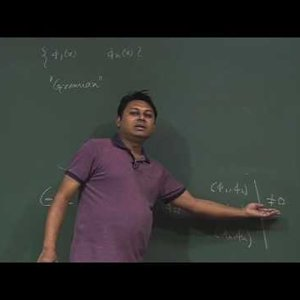### Mathematical Physics 1 by Prof. Samudra Roy (NPTEL):- Lecture 21: Orthogonal functions

• 0
• 0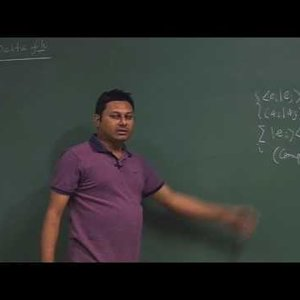### Mathematical Physics 1 by Prof. Samudra Roy (NPTEL):- Lecture 22: Delta Function, Completeness

• 0
• 0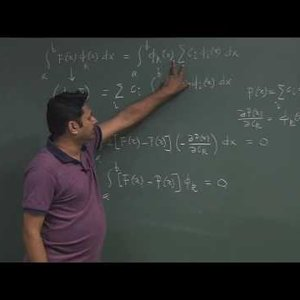### Mathematical Physics 1 by Prof. Samudra Roy (NPTEL):- Lecture 23: Fourier Series

• 0
• 0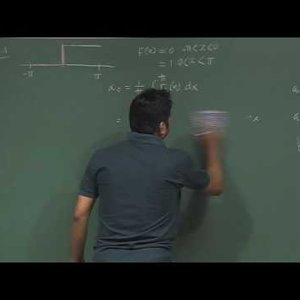### Mathematical Physics 1 by Prof. Samudra Roy (NPTEL):- Lecture 24: Fourier Series (Contd.)

• 0
• 0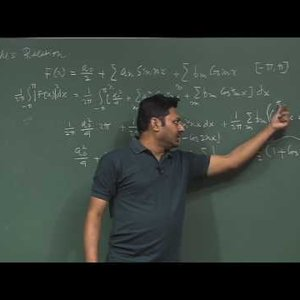### Mathematical Physics 1 by Prof. Samudra Roy (NPTEL):- Lecture 25: Parseval Theorem, Fourier Transform

• 0
• 0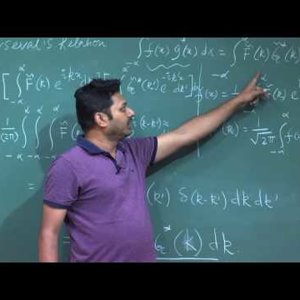### Mathematical Physics 1 by Prof. Samudra Roy (NPTEL):- Lecture 26: Parseval Relation, Convolution Theorem

• 0
• 0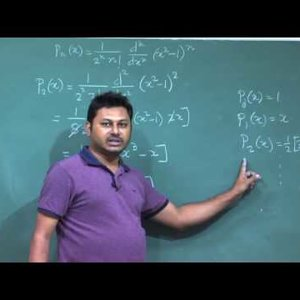### Mathematical Physics 1 by Prof. Samudra Roy (NPTEL):- Lecture 27: Polynomial space, Legendre Polynomial

• 0
• 0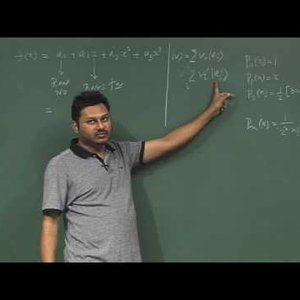### Mathematical Physics 1 by Prof. Samudra Roy (NPTEL):- Lecture 28: Monomial Basis, Factorial Basis, Legendre Basis

• 0
• 0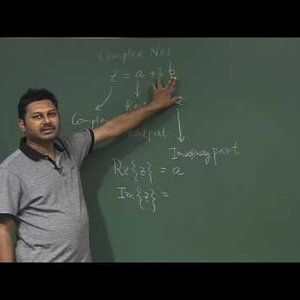### Mathematical Physics 1 by Prof. Samudra Roy (NPTEL):- Lecture 29: Complex Numbers

• 0
• 0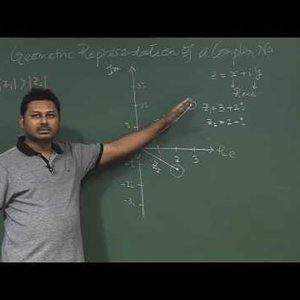### Mathematical Physics 1 by Prof. Samudra Roy (NPTEL):- Lecture 30: Geometrical interpretation of complex numbers

• 0
• 0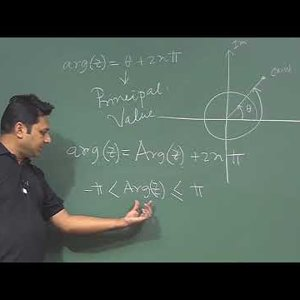### Mathematical Physics 1 by Prof. Samudra Roy (NPTEL):- Lecture 31 : de Moivre’s Theorem

• 0
• 0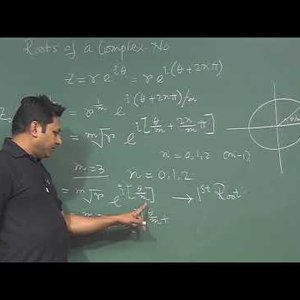### Mathematical Physics 1 by Prof. Samudra Roy (NPTEL):- Lecture 32 : Roots of a complex number

• 0
• 0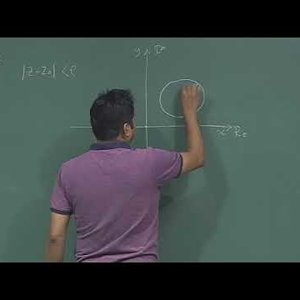### Mathematical Physics 1 by Prof. Samudra Roy (NPTEL):- Lecture 33 : Set of complex no, Stereographic projection

• 0
• 0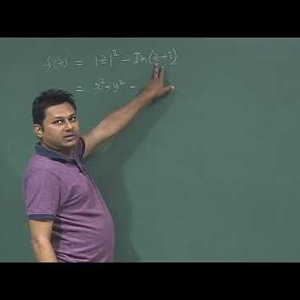### Mathematical Physics 1 by Prof. Samudra Roy (NPTEL):-Lecture 34 : Complex Function, Concept of Limit

• 0
• 0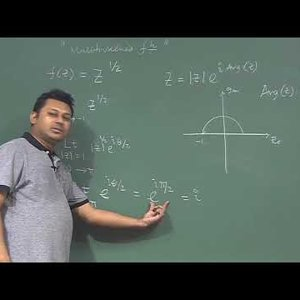### Mathematical Physics 1 by Prof. Samudra Roy (NPTEL):- Lecture 35 : Derivative of Complex Function, Cauchy-Riemann Equation

• 0
• 0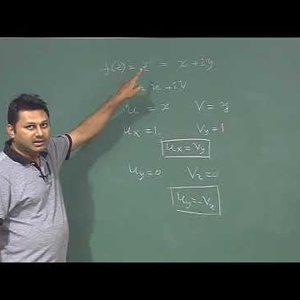### Mathematical Physics 1 by Prof. Samudra Roy (NPTEL):- Lecture 36 : Analytic Function

• 0
• 0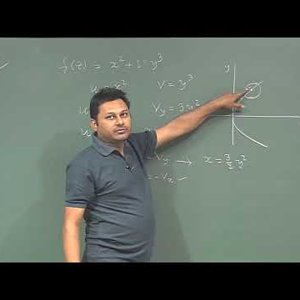### Mathematical Physics 1 by Prof. Samudra Roy (NPTEL):- Lecture 37 : Harmonic Conjugate

• 0
• 0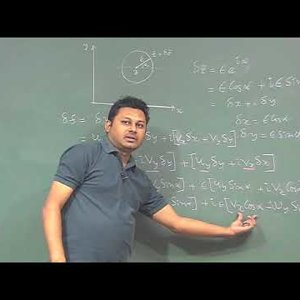### Mathematical Physics 1 by Prof. Samudra Roy (NPTEL):- Lecture 38 : Polar form of Cauchy-Riemann Equation

• 0
• 0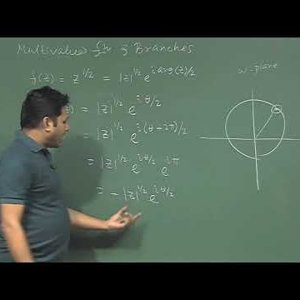### Mathematical Physics 1 by Prof. Samudra Roy (NPTEL):- Lecture 39 : Multi-valued function and Branches

• 0
• 0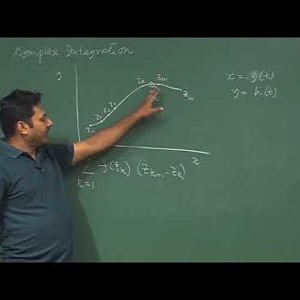### Mathematical Physics 1 by Prof. Samudra Roy (NPTEL):- Lecture 40 : Complex Line Integration, Contour, Regions

• 0
• 0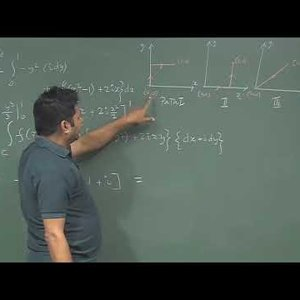### Mathematical Physics 1 by Prof. Samudra Roy (NPTEL):- Lecture 41: Complex Line Integration(Cont.)

• 0
• 0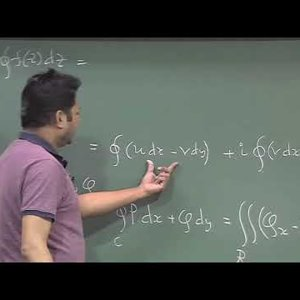### Mathematical Physics 1 by Prof. Samudra Roy (NPTEL):-Lecture 42: Cauchy-Goursat Theorem

• 0
• 0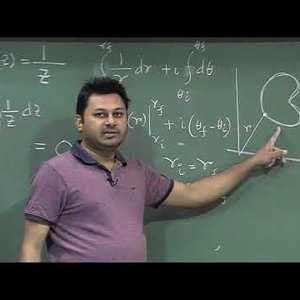### Mathematical Physics 1 by Prof. Samudra Roy (NPTEL):- Lecture 43: Application of Cauchy-Goursat Theorem

• 0
• 0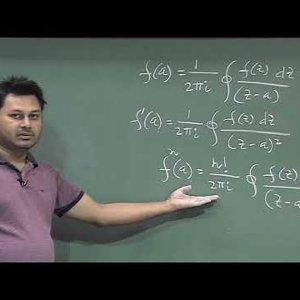### Mathematical Physics 1 by Prof. Samudra Roy (NPTEL):- Lecture 44: Cauchy’s Integral Formula

• 0
• 0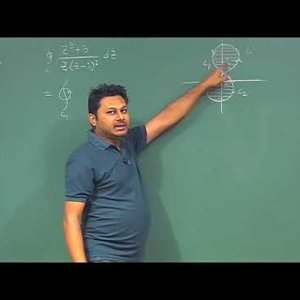### Mathematical Physics 1 by Prof. Samudra Roy (NPTEL):- Lecture 45: Cauchy’s Integral Formula (Contd.)

• 0
• 0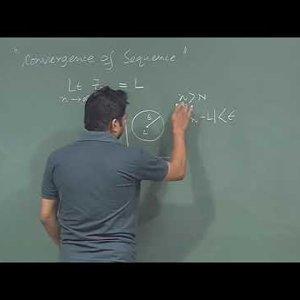### Mathematical Physics 1 by Prof. Samudra Roy (NPTEL):- Lecture 46: Series and Sequence

• 0
• 0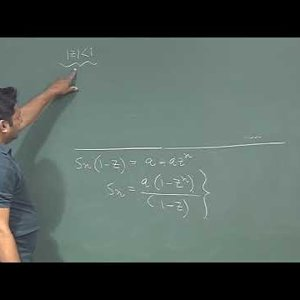### Mathematical Physics 1 by Prof. Samudra Roy (NPTEL):- Lecture 47: Series and Sequence (Contd.)

• 0
• 0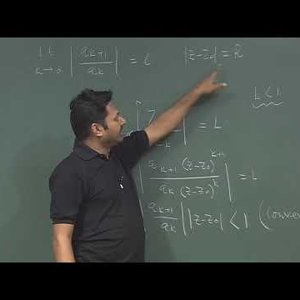### Mathematical Physics 1 by Prof. Samudra Roy (NPTEL):- Lecture 48:Circle and radius of convergence

• 0
• 0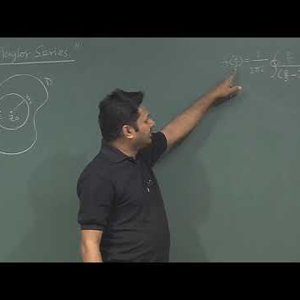### Mathematical Physics 1 by Prof. Samudra Roy (NPTEL):- Lecture 49: Taylor Series

• 0
• 0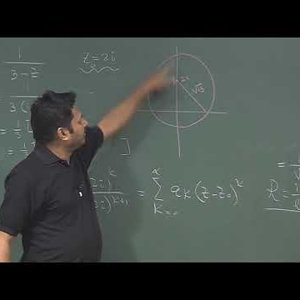### Mathematical Physics 1 by Prof. Samudra Roy (NPTEL):- Lecture 50: Classification of singularity

• 0
• 0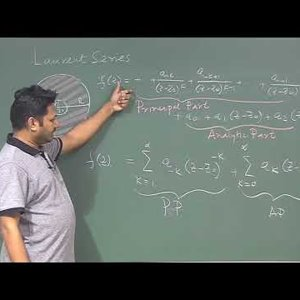### Mathematical Physics 1 by Prof. Samudra Roy (NPTEL):- Lecture 51: Laurent Series, Singularity

• 0
• 0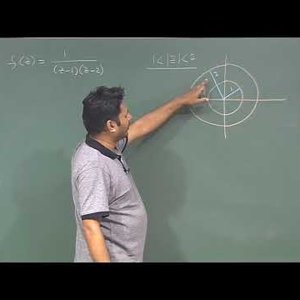### Mathematical Physics 1 by Prof. Samudra Roy (NPTEL):- Lecture 52: Laurent series expansion

• 0
• 0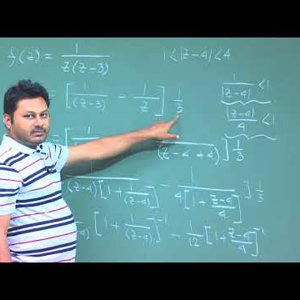### Mathematical Physics 1 by Prof. Samudra Roy (NPTEL):- Lecture 53: Laurent series expansion (Cont), Concept of Residue

• 0
• 0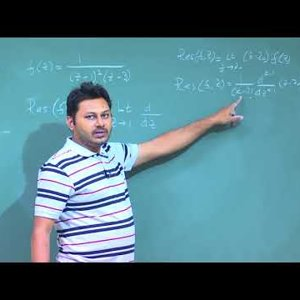### Mathematical Physics 1 by Prof. Samudra Roy (NPTEL):- Lecture 54: Classification of Residue

• 0
• 0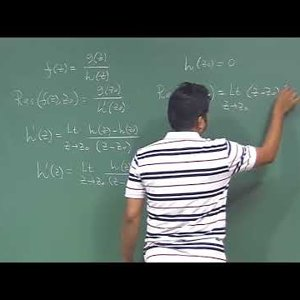### Mathematical Physics 1 by Prof. Samudra Roy (NPTEL):- Lecture 55: Calculation of Residue for quotient

• 0
• 0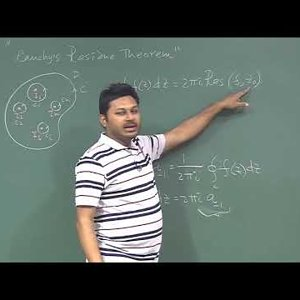### Mathematical Physics 1 by Prof. Samudra Roy (NPTEL):- Lecture 56 : Cauchy’s Residue Theorem

• 0
• 0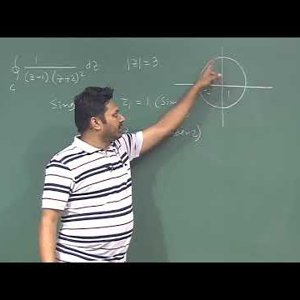### Mathematical Physics 1 by Prof. Samudra Roy (NPTEL):-Lecture 57 : Cauchy’s Residue Theorem (Cont)

• 0
• 0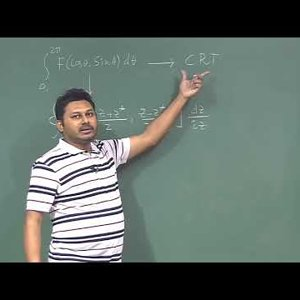### Mathematical Physics 1 by Prof. Samudra Roy (NPTEL):- Lecture 58 : Real Integration using Cauchy’s Residue Theorem

• 0
• 0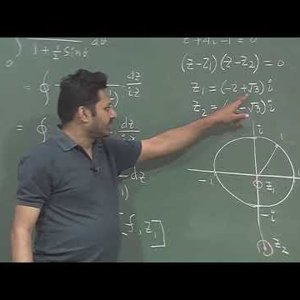### Mathematical Physics 1 by Prof. Samudra Roy (NPTEL):- Lecture 58 : Real Integration using Cauchy’s Residue Theorem (Contd. 1)

• 0
• 0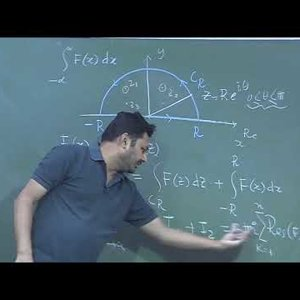### Mathematical Physics 1 by Prof. Samudra Roy (NPTEL):- Lecture 58 : Real Integration using Cauchy’s Residue Theorem (Contd. 2)

• 0
• 0• Marco Fermi
• Dec 2, 2018
• 0
• 0